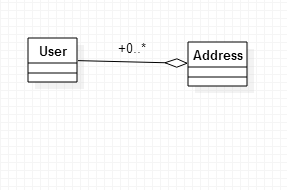## 安装

``pip install SQLAlchemy``

``>>> import sqlalchemy``>>> print sqlsqlalchemy.__version__ ``1.1.0``

## 声明模型

ORM 的一个最大的特点就是有模型，也就是我们常说的 Schema，这个 Scheme 是定义我们模型有什么元素，每个元素是什么类型，元素之间有什么关系的约束，在 SQLAlchemy 中定义模型有两种方式，分别是 经典模式现代模式，这里我就举 现代模式 的例子吧，毕竟 经典模式 还是比较麻烦的。

``from sqlalchemy import Column, String, Integer``from sqlalchemy.ext.declarative import declarative_base``Base = declarative_base()``class  User(Base):``    __tablename__ = 'user'``    id = Column(Integer, primary_key=True)``    name =  Column(String(50))``

``>>> User.__table__``Table('user', MetaData(bind=None), Column('id', Integer(), table=<user>, primary_key=True, nullable=False), Column('name', String(length=50), table=<user>), schema=None)``

``from sqlalchemy import create_engine``from sqlalchemy.orm import sessionmaker``engine = create_engine('mysql+mysqldb://yetship:[email protected]/test?charset=utf8', echo=True)``Session = sessionmaker(bind=engine)``session = Session()``

``User.metadata.create_all(engine)``

## 增删改查

``user = User(name="Tyrael")``session.add(user)``

``>>> print session.new``IdentitySet([<__main__.User object at 0x7fbcf0239cf8>])``

``session.commit()``

``user = User(name="Tyrael")``session.add(user)``session.commit()``

``session.add(User(name="Tyrael"))``

``usr = session.query(User).first()``session.delete(usr)``session.commit()``

``usr = session.query(User).first()``usr.name = "LiSi"``session.add(usr)``session.commit()``

• equals:
``query.filter(User.name == 'ed')``
• not equals:
``query.filter(User.name != 'ed')``
• LIKE:
``query.filter(User.name.like('%ed%'))``
• IN:
``query.filter(User.name.in_(['ed', 'wendy', 'jack']))``# works with query objects too:``query.filter(User.name.in_(``        session.query(User.name).filter(User.name.like('%ed%'))``))``
• NOT IN:
``query.filter(~User.name.in_(['ed', 'wendy', 'jack']))``
• IS NULL:
``query.filter(User.name == None)``# alternatively, if pep8/linters are a concern``query.filter(User.name.is_(None))``
• IS NOT NULL:
``query.filter(User.name != None)``# alternatively, if pep8/linters are a concern``query.filter(User.name.isnot(None))``
• AND:
``# use and_()``from sqlalchemy import and_``query.filter(and_(User.name == 'ed', User.fullname == 'Ed Jones'))``# or send multiple expressions to .filter()``query.filter(User.name == 'ed', User.fullname == 'Ed Jones')``# or chain multiple filter()/filter_by() calls``query.filter(User.name == 'ed').filter(User.fullname == 'Ed Jones')``Note``Make sure you use and_() and not the Python and operator!``
• OR:
``from sqlalchemy import or_``query.filter(or_(User.name == 'ed', User.name == 'wendy'))``Note``Make sure you use or_() and not the Python or operator!``
• MATCH:
``query.filter(User.name.match('wendy'))``

• first()： 使用 limit 选取一个元素
• all()：返回符合条件的所有结果列表
• one()：获取所有符合条件的结果，如果结果数量不是刚好一个都抛出错误
• one_or_none()：和 one 差不多，不过当有多个记录的时候不报错，没有元素的时候返回 None
• scalar()：调用 one，但有数据的时候返回第一列的数据

``session.query(User).filter(User.name.like('%ed')).count()``

``session.query(func.count('*')).select_from(User).scalar()``

## 模型关系

• 一对一
• 一对多（多对一）
• 多对多

• 一对多
• 多对多

• 一种是在表中加外键，这其实就涵盖了一对一多对一 的关系
• 建立一张关联表，表中有两个表的外键，这其实处理的就是 多对多 的关系

### 一对多的关系``#!/usr/bin/env python``# encoding: utf-8``from sqlalchemy import create_engine``from sqlalchemy import Table, Column, Integer, ForeignKey``from sqlalchemy.orm import relationship, sessionmaker``from sqlalchemy.ext.declarative import declarative_base``engine = create_engine('sqlite:///:memory:', echo=True)``Session = sessionmaker(bind=engine)``session = Session()``Base = declarative_base(bind=engine)``class User(Base):``    __tablename__ = 'user'``    id = Column(Integer, primary_key=True)``    addresses = relationship("Address")``class Address(Base):``    __tablename__ = 'address'``    id = Column(Integer, primary_key=True)``    user_id = Column(Integer, ForeignKey('user.id'))``if  __name__ == "__main__":``    Base.metadata.create_all()``    u = User()``    session.add(u)``    session.commit()``    a1 = Address(user_id=u.id)``    a2 = Address(user_id=u.id)``    session.add(a1)``    session.add(a2)``    session.commit()``    print "address: {}".format(u.addresses)``

• ForeignKey：外键，指定了 user_id 和 User 的关系
• relationship：这个就绑定了两个 Model 的联系，可以通过 User 直接得到所有的地址

``class User(Base):``    __tablename__ = 'user'``    id = Column(Integer, primary_key=True)``    addresses = relationship("Address")``class Address(Base):``    __tablename__ = 'address'``    id = Column(Integer, primary_key=True)``    user_id = Column(Integer, ForeignKey('user.id'))``    user = relationship("User")``

``class User(Base):``    __tablename__ = 'user'``    id = Column(Integer, primary_key=True)``    addresses = relationship("Address", backref="user")``class Address(Base):``    __tablename__ = 'address'``    id = Column(Integer, primary_key=True)``    user_id = Column(Integer, ForeignKey('user.id'))``

``class User(Base):``    __tablename__ = 'user'``    id = Column(Integer, primary_key=True)``    addresses = relationship("Address", back_populates="user")``class Address(Base):``    __tablename__ = 'address'``    id = Column(Integer, primary_key=True)``    user_id = Column(Integer, ForeignKey('user.id'))``    user = relationship("User", back_populates="addresses")``

``class User(Base):``    __tablename__ = 'user'``    id = Column(Integer, primary_key=True)``    addresses = relationship("Address")``class Address(Base):``    __tablename__ = 'address'``    id = Column(Integer, primary_key=True)``    user_id = Column(Integer, ForeignKey('user.id'))``    user = relationship("User")``Base.metadata.create_all()``u = User()``a2 = Address()``a2.user = u``print "address: {}".format(u.addresses)``

### 多对多关系

``user_address_table = Table(                         ``    'user_adderss', Base.metadata,                  ``    Column('user_id', Integer, ForeignKey('user.id')),``    Column('address_id', Integer, ForeignKey('address.id'))``)``

``class User(Base):        ``    __tablename__ = 'user'                                  ``    id = Column(Integer, primary_key=True)                  ``    addresses = relationship("Address", secondary=user_address_table)``class Child(Base):       ``    __tablename__ = 'address'                               ``    id = Column(Integer, primary_key=True)                  ``    users = relationship("user", secondary=user_address_table)``

``# 增/查``u1 = User()``u2 = User()``session.add(u1)``session.add(u2)``session.commit()``a1 = Address(users=[u1, u2])``a2 = Address(users=[u1, u2])``session.add(a1)``session.add(a2)``session.commit()``print "children: {}".format(u1.addresses)``print "parent: {}".format(a1.users)``# 改``u1 = User()``u2 = User()``session.add(u1)``session.add(u2)``session.commit()``a2 = Address()``session.add(a2)``session.commit()``a2.users= [u1, u2]``session.commit()``print "parent: {}".format(u1.addresses)``# 删除``u1 = User()``u2 = User()``session.add(u1)``session.add(u2)``session.commit()``a2 = Address()``session.add(a2)``session.commit()``a2.users= [u1, u2]``session.commit()``print "parent: {}".format(u1.addresses)``session.delete(u1)``session.commit()``print "parent: {}".format(a2.users)``print "users: {}".format(session.query(User).count())``